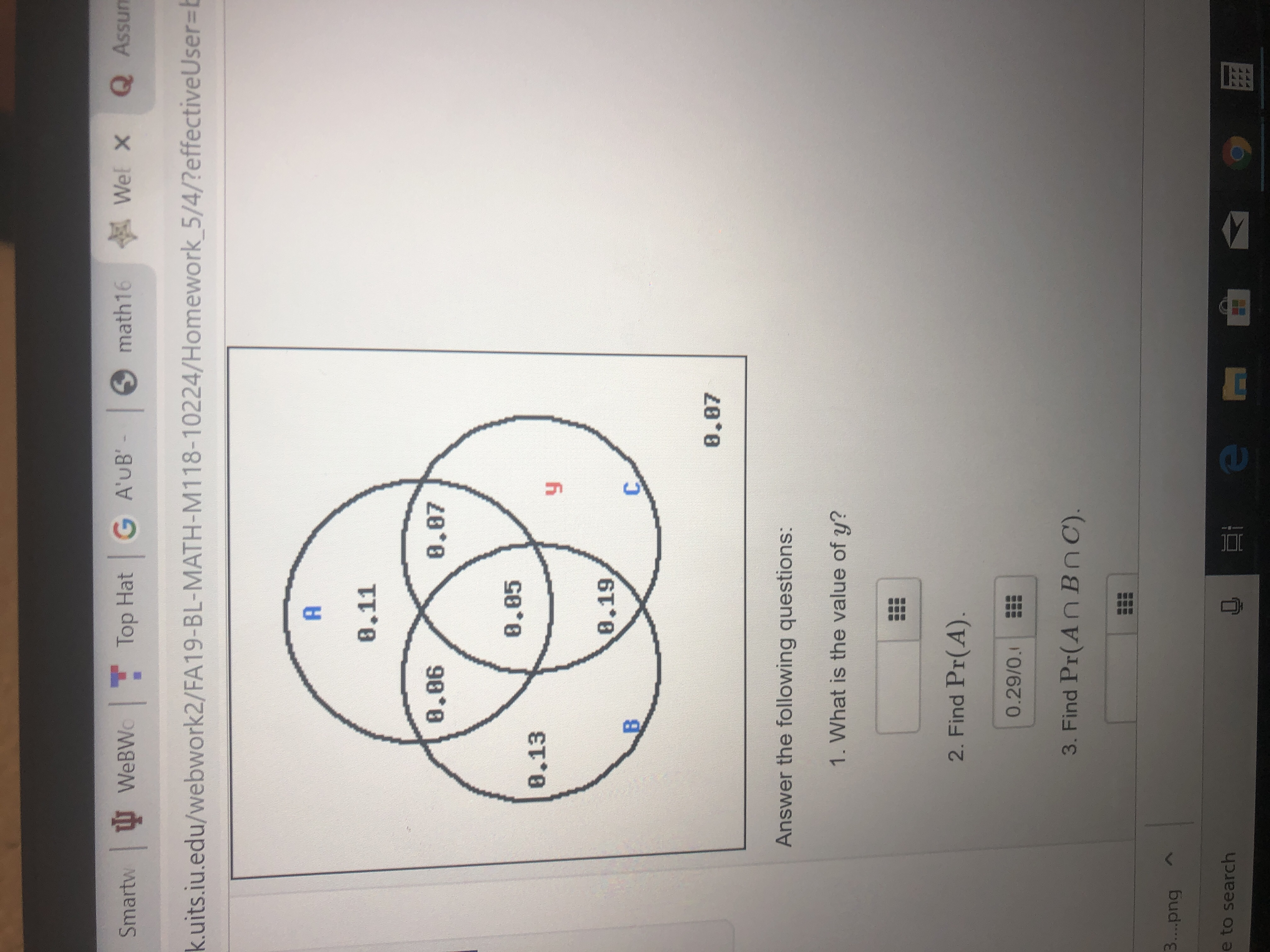# Wel Xmath16Q AssunTop Hat G A'UB'-SmartwWEBWk.uits.iu.edu/webwork2/FA19-BL-MATH-M118-10224/Homework 5/4/?effective User=EA0.118.868.878.058.138.19,BC.8.87Answer the following questions:1. What is the value of y?2. Find Pr(A).0.29/0.3. Find Pr(An BnC)3....pnge to searchusd

Question
9 viewshelp_outlineImage TranscriptioncloseWel X math16 Q Assun Top Hat G A'UB'- Smartw WEBW k.uits.iu.edu/webwork2/FA19-BL-MATH-M118-10224/Homework 5/4/?effective User=E A 0.11 8.86 8.87 8.05 8.13 8.19, B C. 8.87 Answer the following questions: 1. What is the value of y? 2. Find Pr(A). 0.29/0. 3. Find Pr(An BnC) 3....png e to search usd fullscreen
check_circle

Step 1

Use the Venn diagram to determine the required data

Step 2

(1) Now y = 1- sum of the probabilites (disjoint) = 1-(0.11+0.06+0.07+0.05+0.12+0.19+0.07)=0.32

Step 3

(2) P(A) = 0.11+0.06+...

### Want to see the full answer?

See Solution

#### Want to see this answer and more?

Solutions are written by subject experts who are available 24/7. Questions are typically answered within 1 hour.*

See Solution
*Response times may vary by subject and question.
Tagged in

### Math